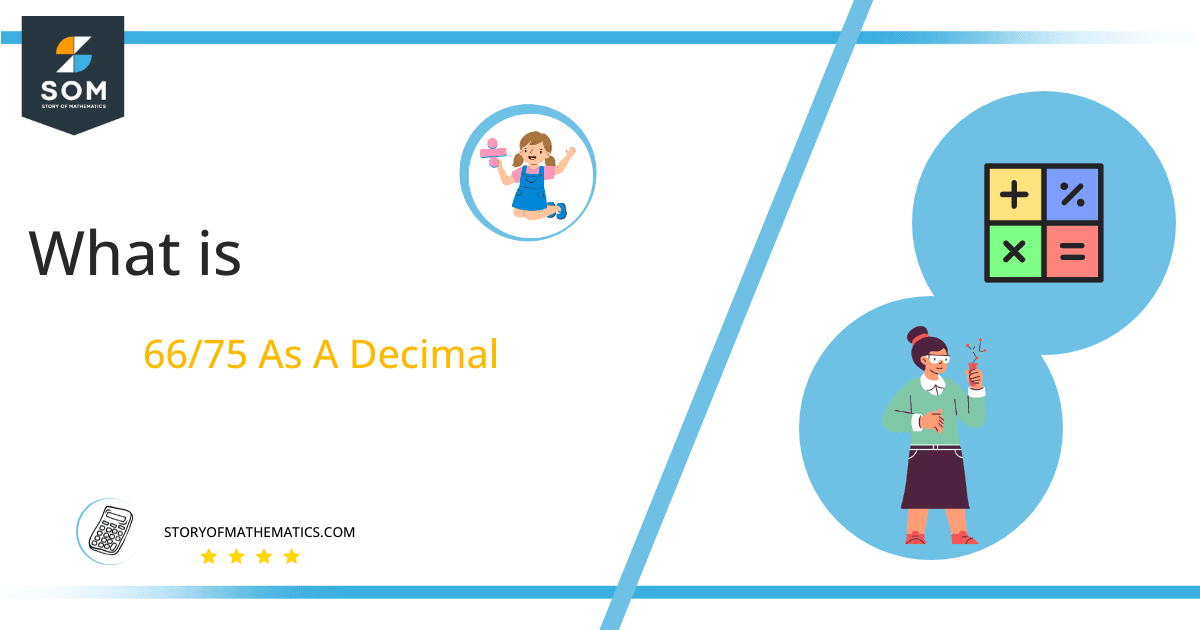# What Is 66/75 as a Decimal + Solution With Free Steps

The fraction 66/75 as a decimal is equal to 0.88.

When we divide a number p by another number q, we usually see this written as p $\boldsymbol\div$ q, where ps is called the dividend and q is called the divisor. Fractions are another way of showing this in the more compact form p/q, where p is the numerator and q is the denominator.Here, we are more interested in the division types that result in a Decimal value, as this can be expressed as a Fraction. We see fractions as a way of showing two numbers having the operation of Division between them that result in a value that lies between two Integers.

Now, we introduce the method used to solve said fraction to decimal conversion, called Long Division, which we will discuss in detail moving forward. So, let’s go through the Solution of fraction 66/75.

## Solution

First, we convert the fraction components, i.e., the numerator and the denominator, and transform them into the division constituents, i.e., the Dividend and the Divisor, respectively.

This can be done as follows:

Dividend = 66

Divisor = 75

Now, we introduce the most important quantity in our division process: the Quotient. The value represents the Solution to our division and can be expressed as having the following relationship with the Division constituents:

Quotient = Dividend $\div$ Divisor = 66 $\div$ 75

This is when we go through the Long Division solution to our problem.Figure 1

## 66/75 Long Division Method

We start solving a problem using the Long Division Method by first taking apart the division’s components and comparing them. As we have 66 and 75, we can see how 66 is Smaller than 75, and to solve this division, we require that 66 be Bigger than 75.

This is done by multiplying the dividend by 10 and checking whether it is bigger than the divisor or not. If so, we calculate the Multiple of the divisor closest to the dividend and subtract it from the Dividend. This produces the Remainder, which we then use as the dividend later.

Now, we begin solving for our dividend 66, which after getting multiplied by 10 becomes 660.

We take this 660 and divide it by 75; this can be done as follows:

660 $\div$ 75 $\approx$ 8

Where:

75 x 8 = 600

This will lead to the generation of a Remainder equal to 660 – 600 = 60. Now this means we have to repeat the process by Converting the 60 into 600 and solving for that:

600 $\div$ 75 = 8

Where:

75 x 8 = 600

Finally, we have a Quotient generated after combining the two pieces of it as 0.88, with a Remainder equal to 0.Images/mathematical drawings are created with GeoGebra.